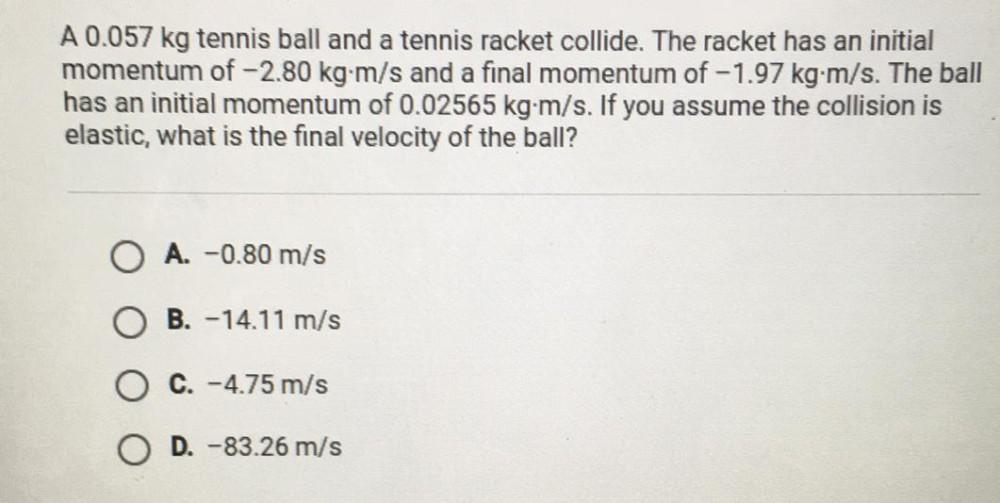Question:

# A 0.057 kg tennis ball and a tennis racket collide. TheA 0.057 kg tennis ball and a tennis racket collide. The racket has an initial momentum of -2.80 kg-m/s and a final momentum of -1.97 kg-m/s. The ball has an initial momentum of 0.02565 kg-m/s. If you assume the collision is elastic, what is the final velocity of the ball? A. -0.80 m/s B. -14.11 m/s C. -4.75 m/s D. -83.26 m/s